# Fraction Rules - Definition with Examples

The Complete K-5 Math Learning Program Built for Your Child

• 40 Million Kids

Loved by kids and parent worldwide

• 50,000 Schools

Trusted by teachers across schools

• Comprehensive Curriculum

Aligned to Common Core

## What is Fraction Rules?

Fraction: A fraction is a part of a whole or a collection and it consists of a numerator and denominator.

Example: If we serve1 part of a cake with 8 equal parts, we have served 18 of the cake.

Let us see how to solve operations involving fractions.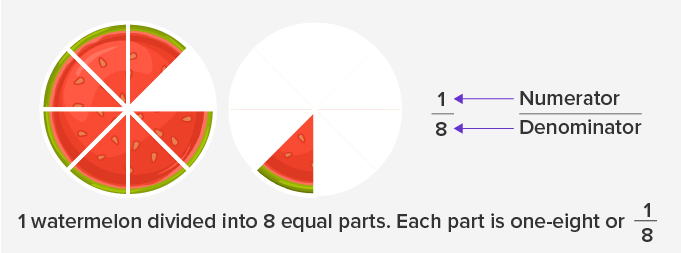## Adding or subtracting fractions with the same denominator

While adding or subtracting two fractions; we need to make sure that the denominators are the same.

Steps:

• Add or subtract the numerators.

• Keep the denominator the same.

• Reduce the answer, if possible.

Example: Solve  114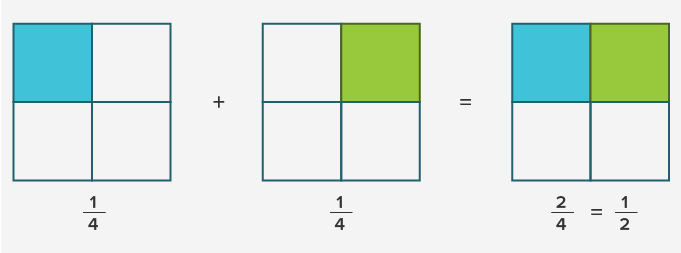Example: Subtract 14 from 34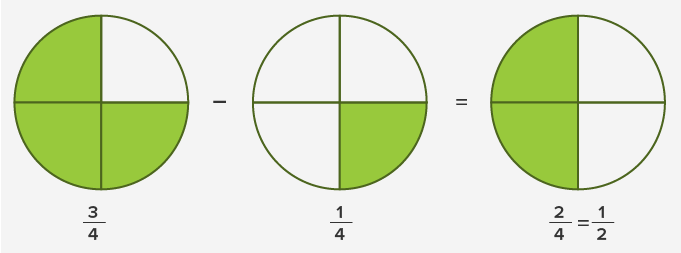## Adding or subtracting fractions with the different denominators:

If the denominators are not the same:

• First, make them the same

• Then add or subtract like fractions with the same denominators.

Example: To solve 14 + 12 , we first make the denominators the same.

We change the denominator 2 and make it 4 by multiplying it by 2. However, we need to multiply the numerator and denominator both by 2 to keep the value of the fraction unchanged.

Multiplying 12 ✕ 22 = 24

Since the denominators are the same we can now add both the fractions.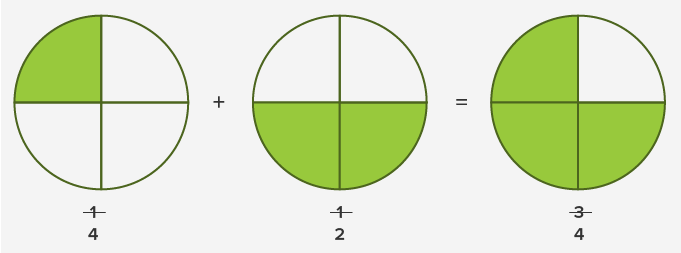Similarly, we use these rules for subtraction.

## Multiplying Fractions

To multiply two fractions we simply multiply the numerators and denominators.

Example:

23 ✕ 315 = ?

First, simplify the fraction 315 to its lowest term.## Dividing Fractions

While dividing two fractions:

• Inverse the second fraction, that is, interchange its numerator and denominator to get the reciprocal.

• Multiply the first fraction by the reciprocal of the second fraction.

Example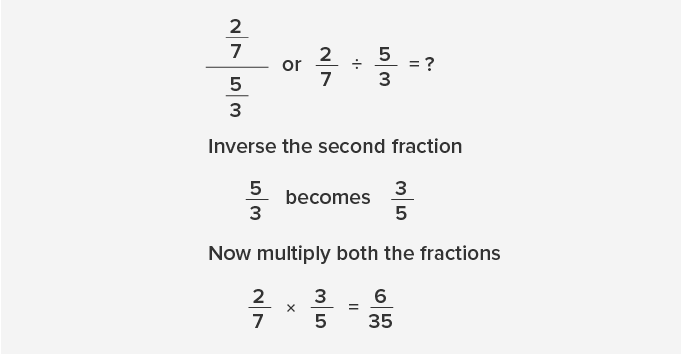## Solving improper fractions:

Fractions with a numerator larger than the denominator are called improper fractions. When we solve improper fractions, the result can be a mixed number (a fraction with a whole number and a proper fraction).

Example

38= ?

• Divide the numerator by the denominator.

38 ÷ 7 = 5 Quotient and 3 Remainder

• Write down the whole number answer.

5

• Then write down the remainder above the denominator.

5 37

Therefore,  387 = 5 37

Thus, by solving an improper fraction 387 we get a mixed number 5 37

 Fun Facts We can multiply two fractions with different denominators, but we cannot add and subtract them.

Won Numerous Awards & Honors